Figures for "Taiji variations"

Cameron Browne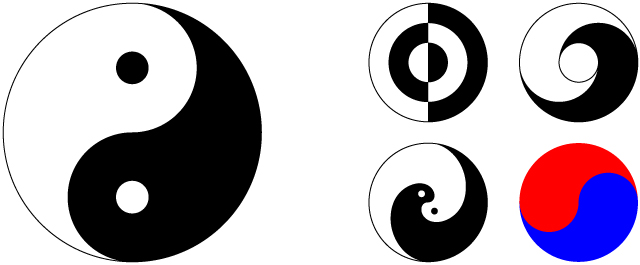Figure 1. The modern taiji (left) and some variations (right).Figure 2. Geometric construction of the figure.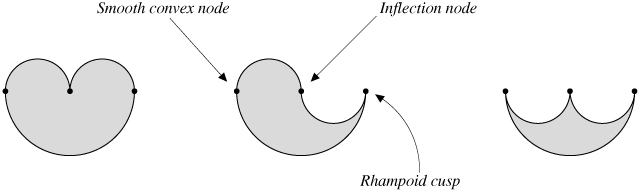Figure 3. The three-piece PC curve types: cardioid, fish, and arbelos.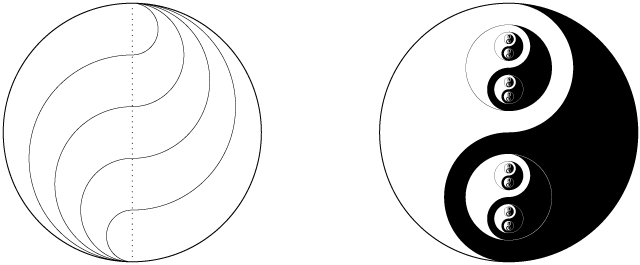Figure 4. Asymmetrical fish fitting (left) and a fractal design (right).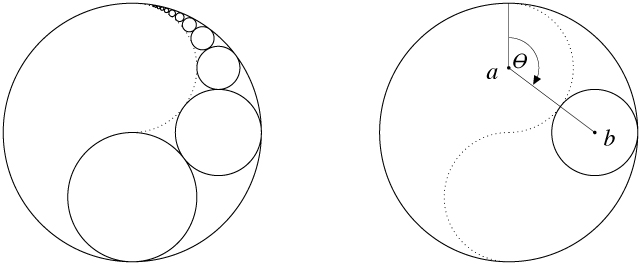Figure 5. Rolling a circle to construct a fish.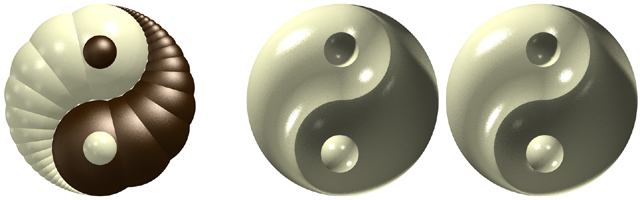Figure 6. Extruded polyspheres (left) and a stereo pair of a carved figure (right).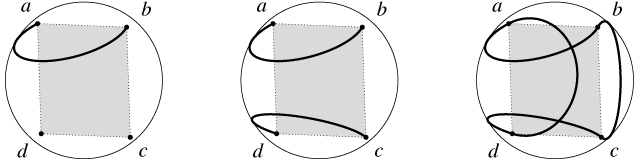Figure 7. Simplified baseball curve from reflections and rotations of a single circular arc.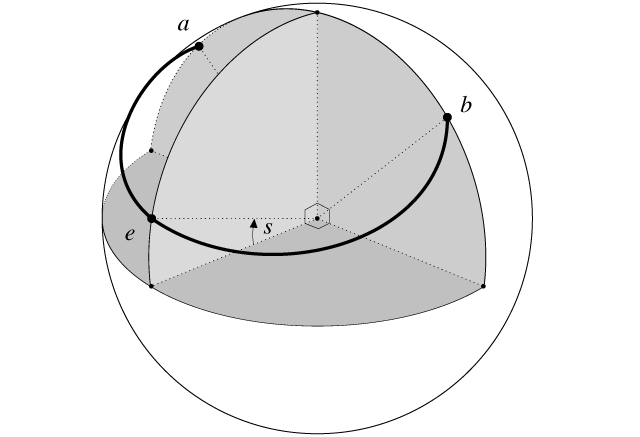Figure 8. Parameterisation by separation angle s.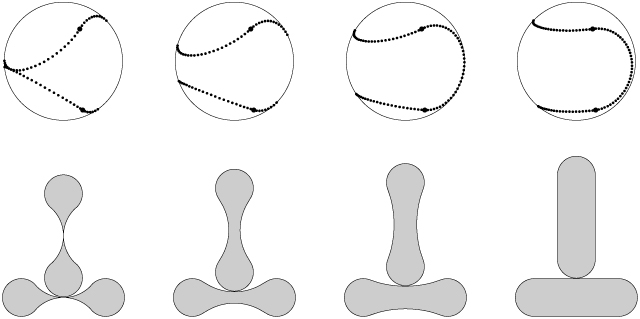Figure 9. Simplified baseball stitching at 0, 15, 30 and 45 degrees of separation, with the unfolded surface patches for each design shown.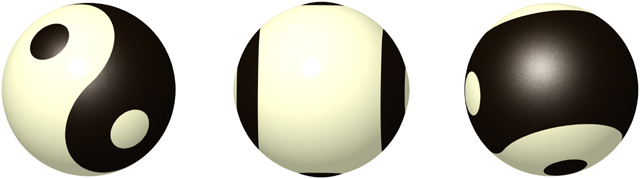Figure 10. The standard taiji ball (s = 45) viewed from the optimal angle (left) and two less interesting angles.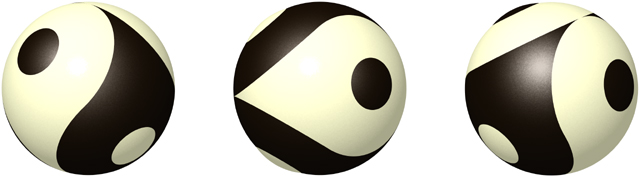Figure 11. The new tailed taiji ball (s = 0).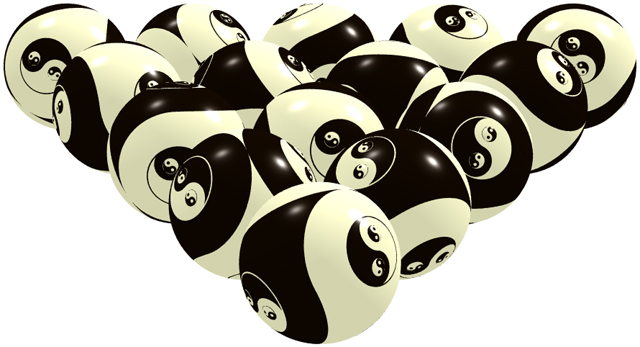Figure 12. A rack of tailed taiji balls with fractal eyes.Figure 13. A klein yang (left) and a false klein pair (right).